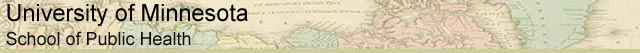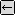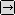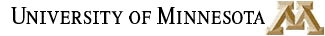### MATH REVIEW: USEFUL MATH FOR EVERYONE

SECTION 4.2. WHAT IS A LOGARITHM?back to Logarithms, Page 1

### Base Ten Logarithms

We saw above that base ten logarithms are expressions in which the number being raised to a power is ten. The base ten log of 1000 is three:

log 1000 = 3

103 = 1000

So far, we've worked with expressions that have whole numbers as solutions. Here's one that does not. What is the log of 4?

log 4 = x

log 4 ≈ 0.602

because

10 0.602 ≈ 4

### Natural Logarithms

Logarithms with a base of 'e' are called natural logarithms. What is 'e'?

'e' is a very special number approximately equal to 2.718. 'e' is a little bit like pi in that it is the result of an equation and it's a big long number that never ends. For those of you who have had calculus, you might remember that ex is special because its derivative is itself. If you want to know more about 'e', check any trigonometry text, such as page 234 of Ruud, W.L. and T.L. Shell. Prelude to Calculus, 2nd ed. 1993. Boston: PWS Publishing Company.

Most scientific calculators have an 'e' button and an 'ln' button, so you don't need to memorize the value of 'e'.to Logarithms, Page 3